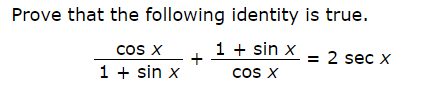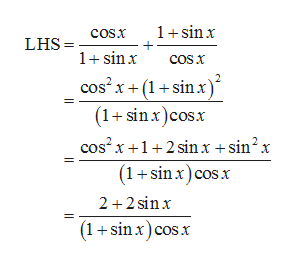# Prove that the following identity is true.1sin xcos X= 2 sec x1sin xcoS X

Question
1 views

Photo is attachedhelp_outlineImage TranscriptioncloseProve that the following identity is true. 1sin x cos X = 2 sec x 1sin x coS X fullscreen
check_circle

Step 1

The given identity is,

Step 2

Consider the LHS of the given equation.help_outlineImage Transcriptionclose1+sinx cosx LHS= 1 sinx cos x cos x (1+sinx) (1+ sinx)cos.x cos2 x+12 sinx + sin2x (1sin x) cos x 2 2 sinx (1+sin x) cos.x fullscreen
Step 3

Further simplify the equ...

### Want to see the full answer?

See Solution

#### Want to see this answer and more?

Solutions are written by subject experts who are available 24/7. Questions are typically answered within 1 hour.*

See Solution
*Response times may vary by subject and question.
Tagged in
MathTrigonometry

### Trigonometric Ratios